# Distance formula – Coordinate Geometry | Class 10 Maths

The distance formula is one of the important concepts in coordinate geometry which is used widely. By using the distance formula we can find the shortest distance i.e drawing a straight line between points. There are two ways to find the distance between points:

1. Pythagorean theorem
2. Distance formula

## Pythagorean Theorem

This theorem is similar to the Pythagoras theorem but the use of it here is a little different. Normally by Pythagoras theorem, we will find the missing length in the right triangle. Here also we do the same thing but before that, we have to find the coordinates of the triangle.

Suppose from a point boy walks 4 m to the west and took a turn to the south and walked 3 m. Now to calculate the shortest distance from the initial point to the final point would be the hypotenuse of the formed triangle as shown below.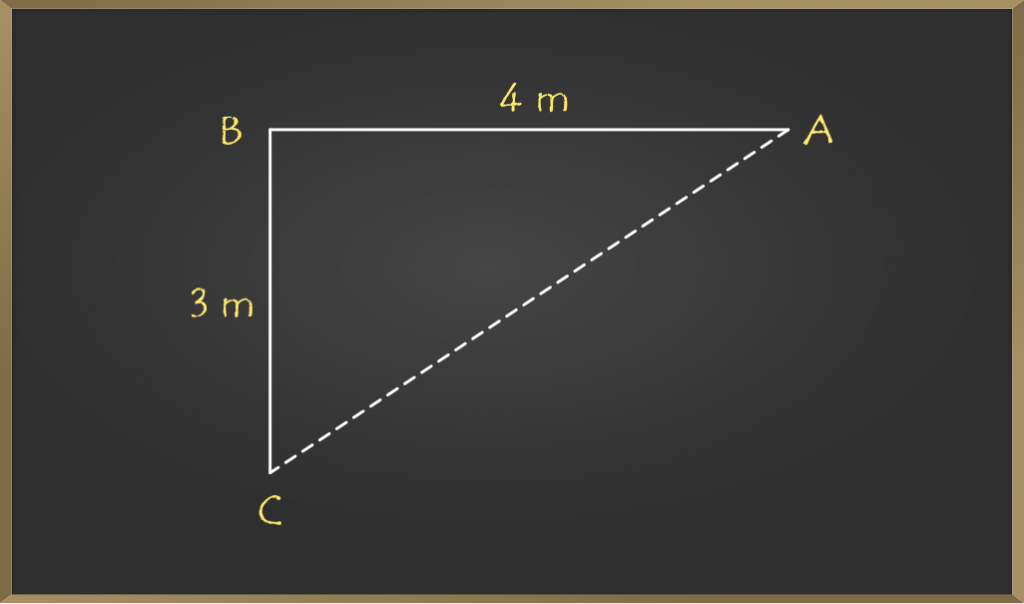In the above diagram as you can see the initial and final points are A and C respectively. The distance is given between points A, B is 4 m and between points B, C is 3 m. To find the shortest distance which is nothing but AC we will use the Pythagorean theorem.

This is calculated using the Pythagoras theorem as follows:

=> AC2 = AB2 + BC2

=> AC = √(16 + 9)

=> AC = √25 = 5 m

Hence the shortest distance is 5 m.

### Sample Problems on Pythagorean Theorem

Problem 1: Using the Pythagorean theorem, find the distance between points (-5, 2) and (7, 2)?

Solution: Let’s try to visualize the coordinates on the graph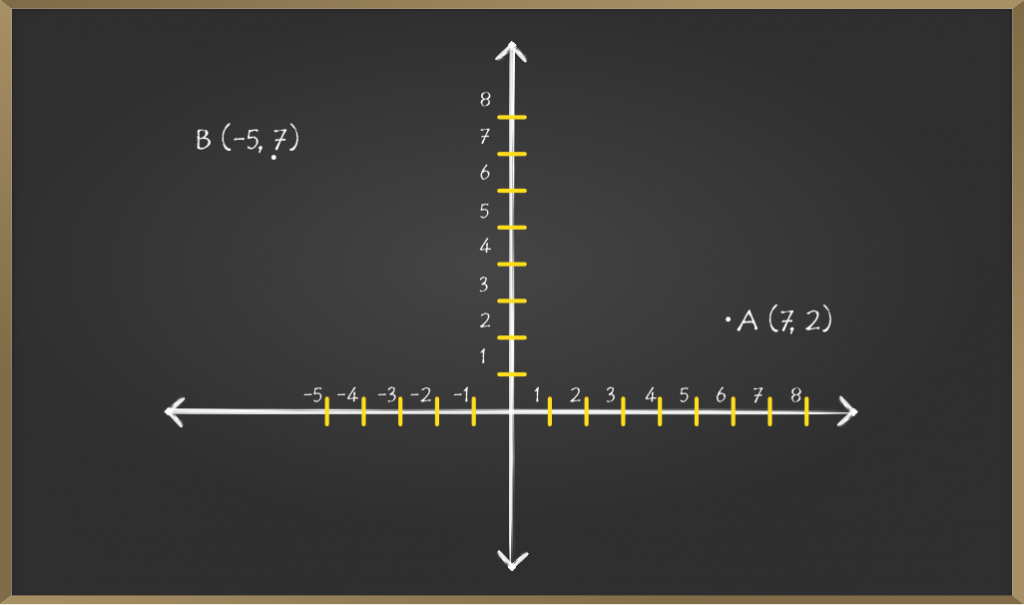Now, let’s join point A and B with a straight line and also draw the horizontal and vertical lines from points A and B respectively.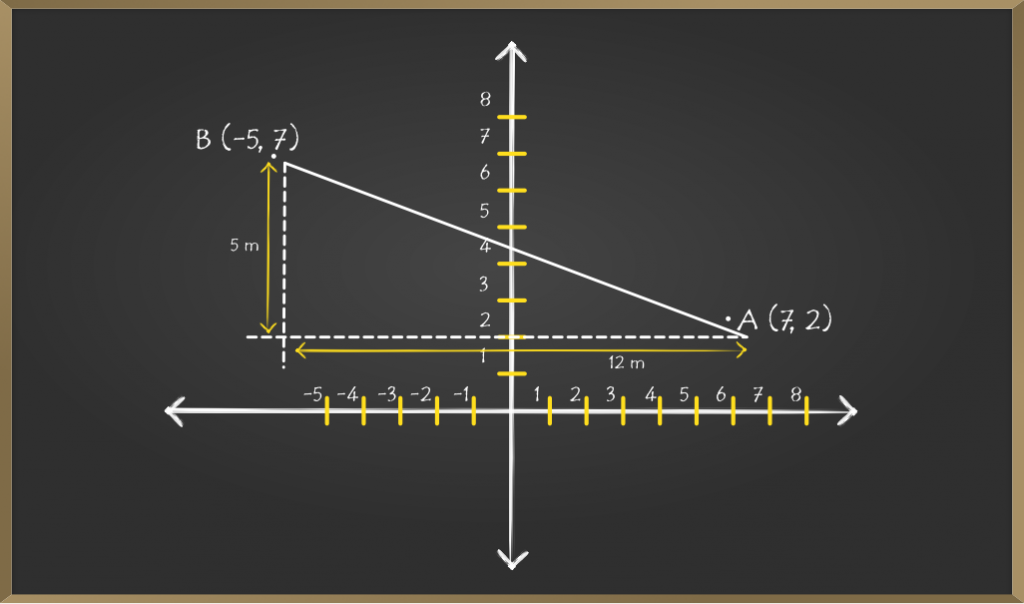Horizontal and vertical lines coincide at point C. Now we have to find the horizontal and vertical distance i.e length of AC and BC.

=> AC = (x2 – x1) = (7 – (-5)) = 12

=> BC = (y2 – y1) = (2 – 7) = -5     [As distance can’t be negative, we have to only consider numerical value]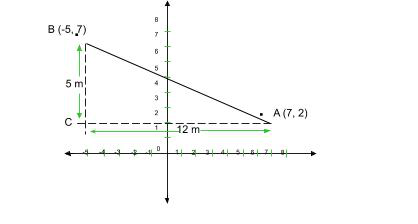As you see in the above diagram, now the problem is turned into basic Pythagoras theorem

=> AB2 = AC2 + BC2

=> AB2 = 52 + 122

=> AB = √(25 + 144)

=> AB = √169 = 13 square units

Therefore, the distance between the given points is 13 sq units.

Problem 2: Using the Pythagorean theorem, find the distance between points (4, 8) and (6, 4)?

Solution: The first thing you have to do after seeing the question is to draw a diagram. Sometimes drawing makes the problem simple.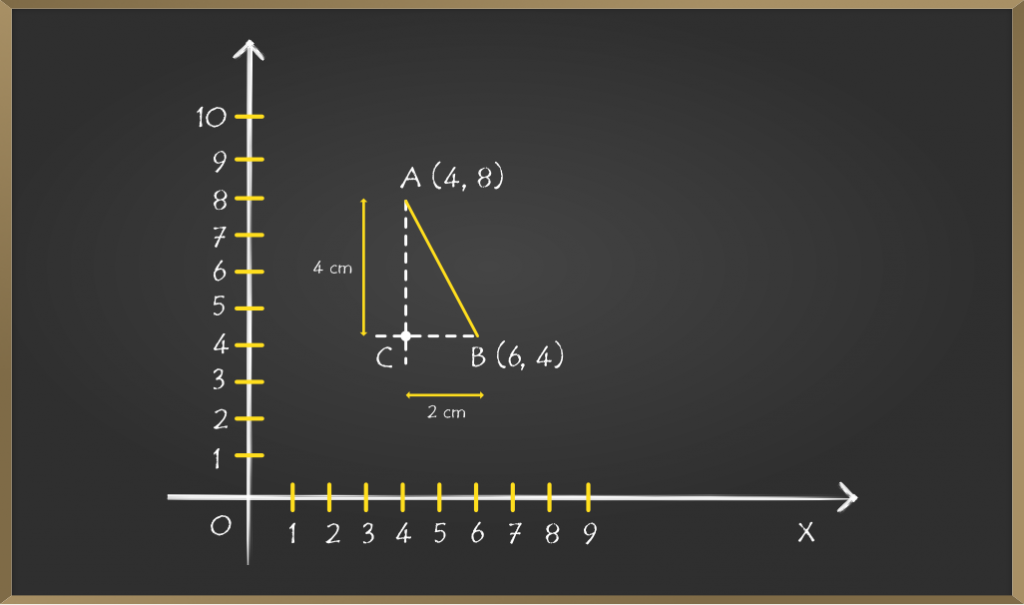As we have to find the distance between points A and B, so first join those points then from point A draw a vertical line and from point B draw a horizontal line and let the point where both extended lines meet be C.

Now to find the coordinates of point C, we should keenly observe that point C is at the same level as point B i.e the Y coordinate will be the same, and similarly point A and point C will have the same X coordinate.

So coordinates of AC will become (4, 4)

Length of line BC will be (6 – 4) = 2 cm

Length of line AC will be (8 – 4) = 4 cm

Now by using Pythagoras theorem,

=> AB2 = AC2 + BC2

=> AB2 = 42 + 22

=> AB = √(16 + 4)

=> AB = √20 = 4.47 (approx)

Hence the distance between point A and B is 4.47 cm

Problem 3: Given the distance between the points M (x, 2) and N (2, 5) is 5 cm. Find the value of x?

Solution: The first step is to draw the diagram for a clear understanding of the problem.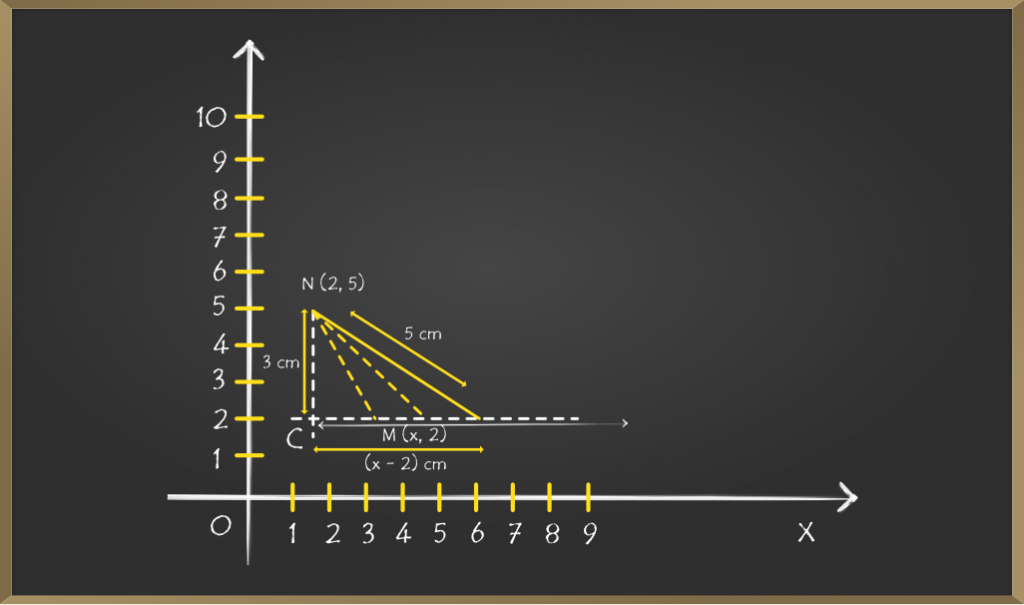This problem is different from previous problems because here we have to find x coordinate of point M where distance is given between the points MN

Point N is fixed but M is not fixed. But we know y coordinate of point M.

So we will draw a horizontal at y = 2. But we don’t know the x coordinate we will have several possibilities.

Now draw a vertical line from point N and name point C where both lines meet.

Length of NC = (5 – 2) = 3 cm

Length of MC = (x – 2) cm

And it is already given NM = 5 cm

Applying Pythagoras theorem,

=> NM2 = NC2 + MC2

=> 52 = 32 + (x – 2)2

=> 25 – 9 = (x – 2)2

=> 16 = (4)2 = (x – 2)2

After cancelling squares on both sides

=> x – 2 = 4

=> x = 6

Therefore value of x is 6 and point M is (6, 2)

## Distance Formula

The distance formula is used to find the distance between any two given points. By Pythagoras theorem, we can derive the distance formula. Using distance formula is much easier than the Pythagorean theorem.

```AB = √[(x2 - x1)² + (y2 - y1)²]

where points are A(x1, y1) and B(x2, y2)
```

Let us look at how this formula is derived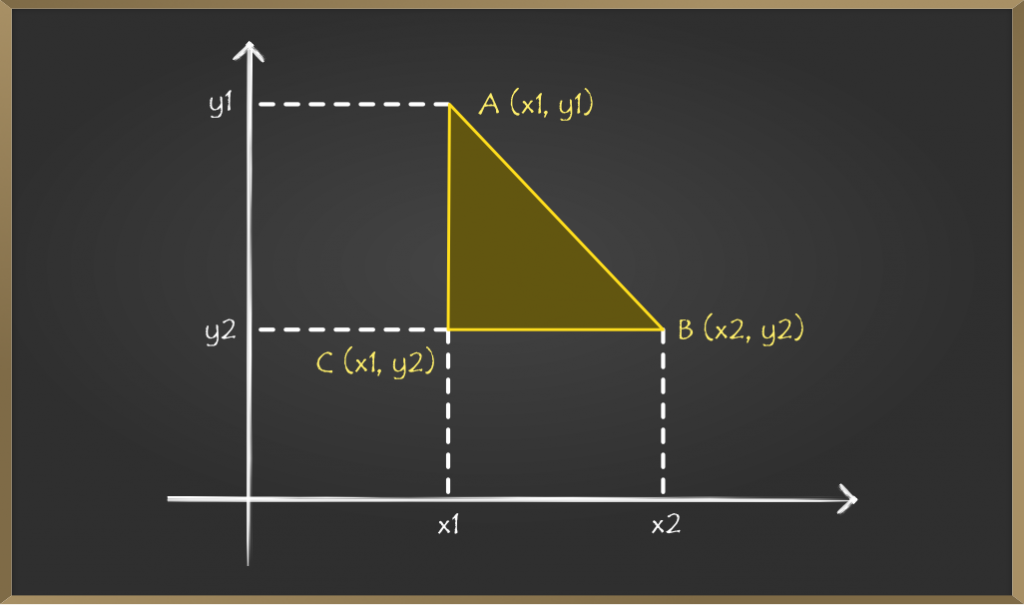In the above diagram, there are 2 points A(x1, y1) and B(x2, y2) for which we have to find the distance between them. Join the points A and B with a straight line. Now draw horizontal lines from points A and B, and they both meet at point C.

Now the coordinates of point C will become C (x1, y2).

Now it looks like a right triangle ACB where side AC is the perpendicular, CB is the base, and AB is the hypotenuse.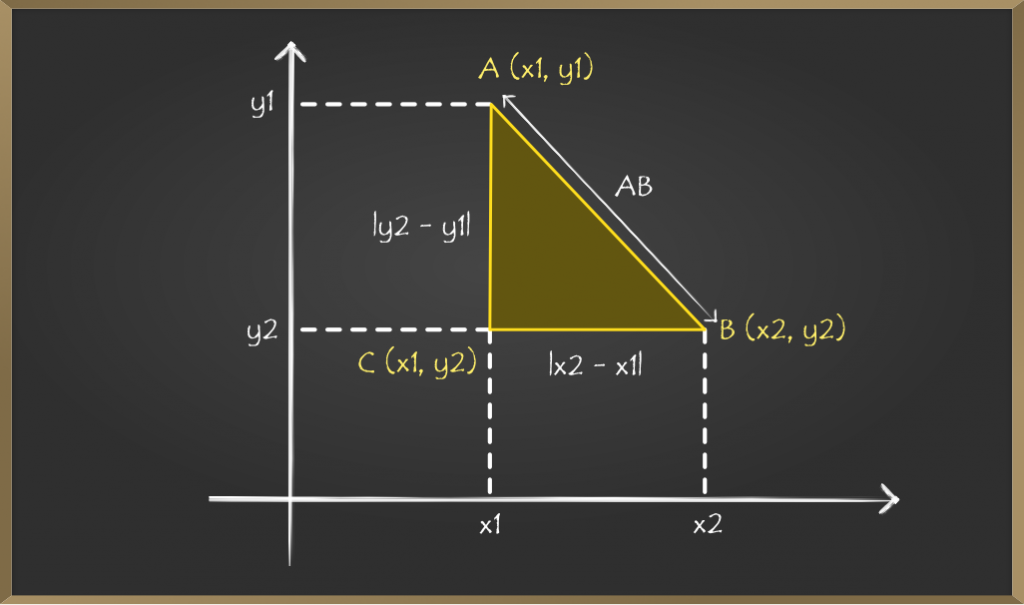Now we need to find the distance between points A and C. Now by seeing the diagram, the distance between them will be the difference between their y coordinate.

=> AC = y2 – y1        …….(1)

Similarly, the distance between points C and B will be the difference between x coordinates.

=> CB = x2 – x1       ……..(2)

ACB is a right-angled triangle. We need to find hypotenuse AB, by Pythagoras theorem

=> AB² = BC² + AC²

From (1) and (2),

=> AB² = (x2 – x1)² + (y2 – y1)²

Taking Square roots on both sides,

=> AB = √{(x2 – x 1)² + (y2 – y1)²}

So the Distance Formula is,

AB = √[(x2 – x1)² + (y2 – y1)²]

### Sample Problems on Distance Formula

Problem 1: The coordinates of point A are (-4, 0) and the coordinates of point B are (0, 3). Find the distance between these two points.

Solution:

The coordinates of A are = (-4, 0)

The coordinates of B are = (0, 3)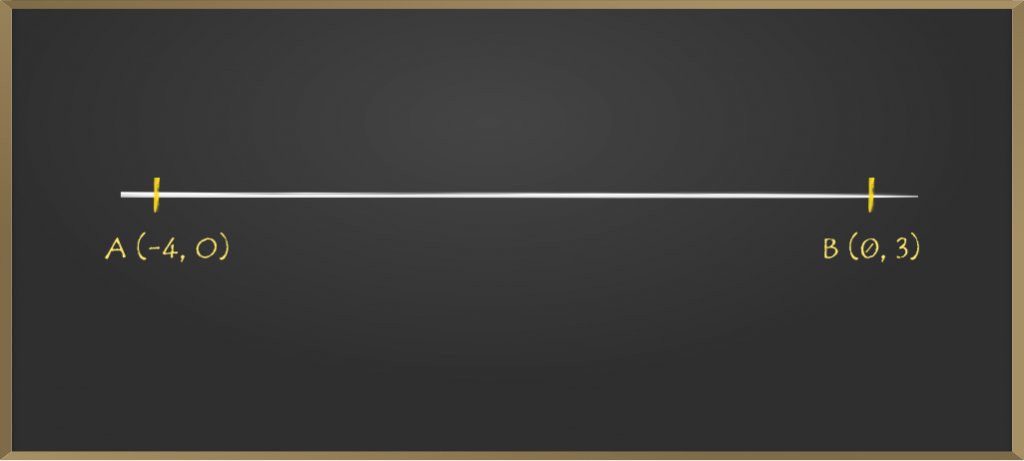Let coordinates of A and B are (x1, y1) and (x2, y2) respectively

By Distance Formula,

=> AB = √[(x2 – x1)² + (y2 – y1)²] = √[{0 – (-4)}²+ (3 – 0)²]

=> √(4² + 3²) = √(16 + 9) = √25

=> 5 units

Therefore the distance between points A and B is 5 units.

Problem 2: Find the distance between the points (7, 3) and (8, 9).

Solution:

Let the coordinates of P = (7, 3) = (x1, y1)

Let the coordinates of Q = (8, 9) = (x2, y2)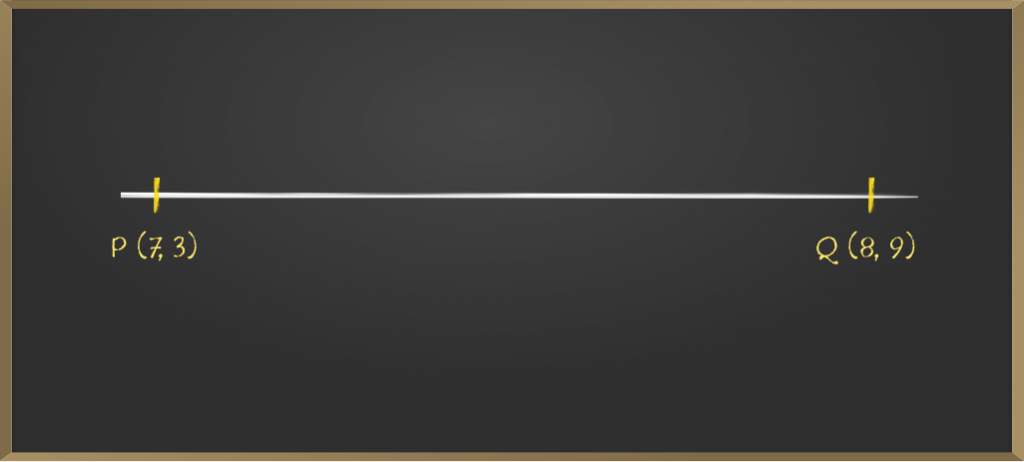By Distance Formula,

=> PQ = √[(x2 – x1)² + (y2 – y1)²]

=> PQ = √[(8 – 7)² + (9 – 3)²]

=> PQ = √(1 + 62

=> PQ = √37 = 6.08 cm  (approx)

Therefore the distance between points P and Q is 6.08 cm.

Problem 3: If the distance between the points (x, 10) and (1, 5) is 13 cm then find the value of x.

Solution:

Let the coordinates of M = (x, 10) = (x1, y1)

Let the coordinates of N = (1, 5) = (x2, y2)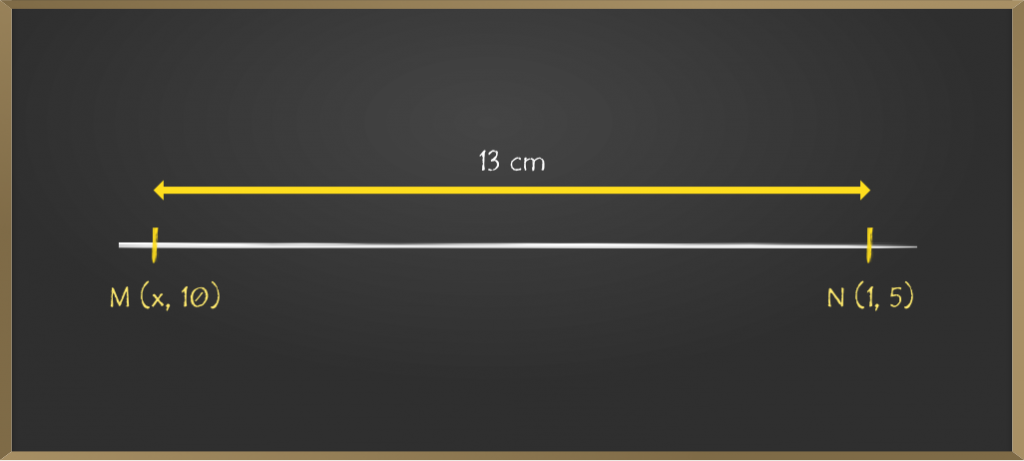By Distance Formula,

=> MN = √[(x2 – x1)² + (y2 – y1)²]

=> 13 = √[(1 – x)² + (5 – 10)²]

(squaring on both sides)

=> 169 = (1 – x)2  + 25

=> (1 – x)2 = 144

=> 1 – x = √144

=> x = 13 or x = -11

Here we can 2 values of x, they are 13 and -11

Whether you're preparing for your first job interview or aiming to upskill in this ever-evolving tech landscape, GeeksforGeeks Courses are your key to success. We provide top-quality content at affordable prices, all geared towards accelerating your growth in a time-bound manner. Join the millions we've already empowered, and we're here to do the same for you. Don't miss out - check it out now!

Previous
Next